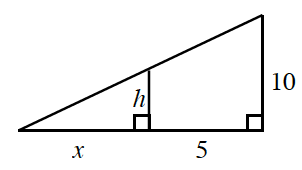### Home > PC3 > Chapter 4 > Lesson 4.2.2 > Problem4-89

4-89.Review the diagram at right.

1. Write an equation for $h$ in terms of $x$.

Use similar triangles. It might help to draw the triangles separately before writing your equation.

2. If $h=3.2$, what is the value of $x$?